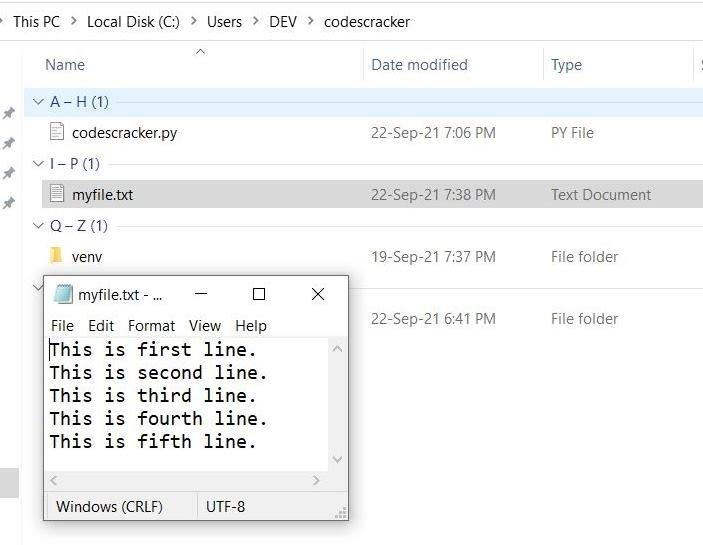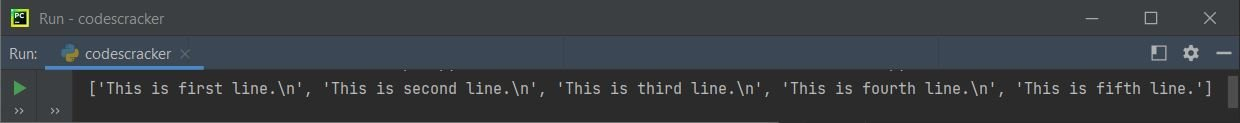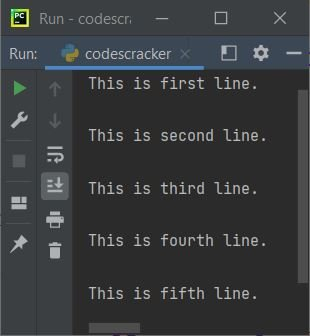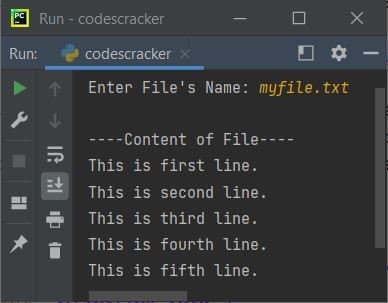Unlike readline(), the readlines() function in Python, used to return all the lines of file at once. That is, all the lines of the file gets returned using readlines() function.

All returned lines using readlines(), will be in the form of list. Where each element is a line. That is, the first element will be the first line of the file, second element will be the second line of the file, and so on.

The syntax to use readlines() function in Python is:

`fo.readlines(size_hint)`

where fo is the file handler or object. The size_hint parameter is optional. This parameter is used to limit the number of lines to be return.

Note: If the total number of bytes returned, exceed the size_hint parameter's value, then no more lines will be returned.

Before creating a program as an example of readlines() function in Python, let's first create a file say myfile.txt inside the current directory with some contents say 5 lines of content. Here is the snapshot shows the current directory, as well as the opened newly created file myfile.txt:Now let's create a program that uses readlines() to read all lines from this file:

```fo = open("myfile.txt", "r")
print(mylines)```

The output produced by this Python program, is shown in the snapshot given below:See the output. It is in the form of list like shown below:

`['This is first line.\n', 'This is second line.\n', 'This is third line.\n', 'This is fourth line.\n', 'This is fifth line.']`

To read the content of file, line by line, then we need to fetch and print the element, one by one, like shown in the program given below:

```fo = open("myfile.txt", "r")

tot = len(mylines)
for i in range(tot):
print(mylines[i])```

Now the output produced by this Python program looks like:See the double newlines between every element or line. It is because, one newline using the default behavior of print() and the another because of last character of every element or line, that is \n, indirectly a newline. Therefore, we need to modify the following statement, from above program:

`print(mylines[i])`

with the statement given below:

`print(mylines[i], end="")`

Now let's modify the above program with a program that receives the name of file from user at run-time of the program. Also this program handles errors when user enters a file that does not exist:

```print("Enter File's Name: ", end="")
fn = input()
try:
fo = open(fn, "r")
print("\n----Content of File----")
for i in range(len(ml)):
print(ml[i], end="")
except FileNotFoundError:

Here is its sample run with same file name as of previous program, that is myfile.txt:Note: The end= parameter is used to change the default behavior of print(). To learn in detail, refer to its separate tutorial.

## Python readlines() With size_hint Parameter

Now let's create another program that uses the optional parameter of readlines() to read the limited number of lines:

```fo = open("myfile.txt", "r")

The output produced by above program will be:

`['This is first line.\n']`

Here is another program:

```fo = open("myfile.txt", "r")

This time, the output produced by above program will be:

`['This is first line.\n', 'This is second line.\n']`

See, when the number of bytes crosses the total bytes/characters available in the current line, the next line too gets printed along with current line.

For example, if there are 10 lines, each line contains 30 characters including newline character. Therefore, providing anything from 1 to 29 prints first line. Providing anything from 30 to 59 prints first and second line. Providing anything from 60 to 89 prints first three lines and so on.

Python Online Test

« Previous Function Next Function »